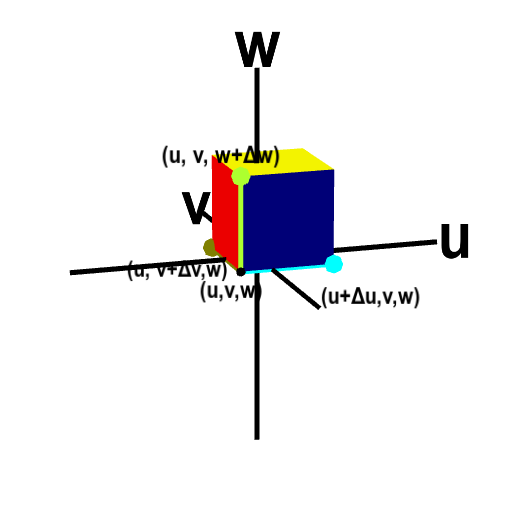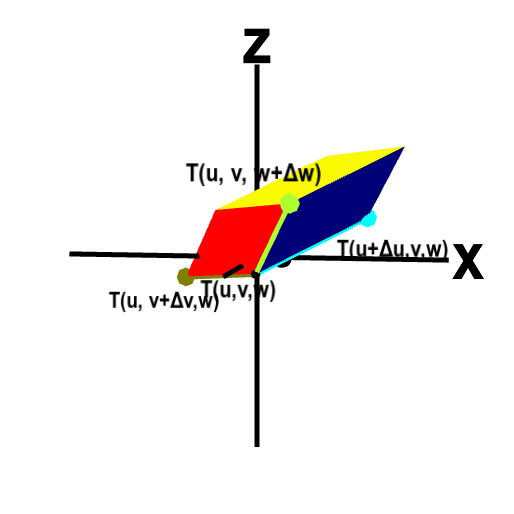# Math Insight

### Applet: Parallelepiped approximation underlying volume transformation calculationThe change of variables function $\cvarf$ transforms a small box (left) in $\cvarfv\cvarsv\cvartv$-space to a curvy region in $xyz$ space. But, since we view the dimensions $\Delta\cvarfv \times \Delta\cvarsv \times \Delta\cvartv$ as being small, we can approximate the image of the box as the shown parallelepiped (right). Four corners around $(\cvarfv,\cvarsv,\cvartv)$ of the box and the corresponding points on the parallelepiped are labeled (though the dimensions $\Delta\cvarfv, \Delta\cvarsv, \Delta\cvartv$ are replaced by $d\cvarfv, d\cvarsv, d\cvartv$). To help visualize the correspondence between the box and the parallelepiped under the map $\cvarf$, the edges between the labeled corners are highlighted with different colors. Since the image of the box is approximated as a parallelepiped, we can easily calculate its volume using the scalar triple product. Drag the image with your mouse to rotate it and better visualize the mapping.